﻿ Calculating the Average Processing Times of the Simulation
QPR Knowledge Base 2017.1

Calculating the Average Processing Times of the Simulation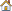Modeling Client Training Guide > Resources and Simulation > Measures and Analysis:

Calculating the Average Processing Times of the SimulationCalculating the Average Processing Times of the Simulation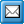When the analysis sheet contains the correct data, actual calculations can be made. The measure values can be shown normally or cumulatively by pressing down the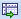Cumulative Calculation button in the ribbon. The cumulative state sums the measure values from left to right so that the rightmost column shows the total sum for the case. The cumulative calculation is available only for single value measures.

The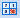Ignore Zero Values button and the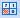Ignore Missing Values button are enabled when a measure is selected. When either button is selected (i.e. pressed down), then the measure value is automatically recalculated with the selected setting (i.e. ignore zero values/missing values). These settings can also be defined for a certain Measure in the Measure Properties dialog.

Also the smallest and greatest total values can be calculated and the average and sum for the cases in the spreadsheet. These values are shown as rows below the other cases. The following buttons can be used for the calculations: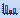Smallest total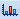Greatest total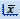Average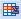Sum of columns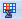Sum of rows

QPR Modeling Client can calculate the Critical path for the selected measure and case. The critical path is the path with the greatest total of measure values summed along the path. The process steps and flows in the path are selected as columns for the spreadsheet. If a process step or flow does not have measure values input for the selected case and measure, it is ignored in the calculations, and is not selected as a column, even if it was otherwise in the path.

The critical path cannot be calculated if no measure values have been input, or the process does not have a starting point for a path, that is a process step with no incoming flows or a flow that starts from scratch. The critical path can be calculated for single value measures only.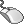Exercise 92: Calculate the average processing time of the simulation.

Note that you need to have run a simulation before doing this exercise and the spreadsheet for the simulation data must be formatted.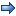1. On the Analysis tab of the ribbon, select Processing Time from the Measure drop-down menu, then select theSum of columns and theAverage buttons to solve the average processing time of the cases. The average processing time can now be found in the lower right corner of the spreadsheet.

See the Analysis topic in QPR Modeling Client - User's Guide for more information.